Related Articles
Manipulating matrices in Julia
• Last Updated : 22 Apr, 2020

Matrices in Julia are the heterogeneous type of containers and hence, they can hold elements of any data type. It is not mandatory to define the data type of a matrix before assigning the elements to the matrix. Julia automatically decides the data type of the matrix by analyzing the values assigned to it. Because of the ordered nature of a matrix, it makes it easier to perform operations on its values based on their index.

Following are some common matrix manipulation operations in Julia:

• Transpose of a matrix
• Flipping a matrix
• Concatenating matrices
• Reshaping a matrix
• Inverse of a matrix

#### Creating a matrix

Julia provides a very simple notation to create matrices. A matrix can be created using the following notation: A = [1 2 3; 4 5 6]. Spaces separate entries in a row and semicolons separate rows. We can also get the size of a matrix using size(A).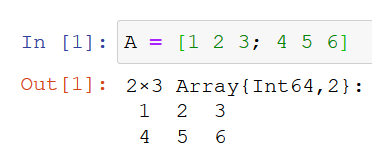#### Transpose of a matrix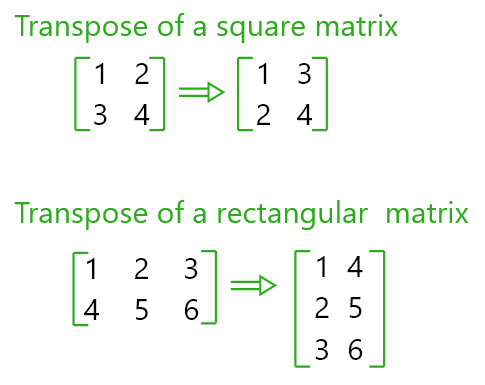• The transpose operation flips the matrix over its diagonal by switching the rows and columns.
• Let A be a matrix. We can get the transpose of A by using A’.
• Example 1:

 `# Defining a square matrix of size (2, 2) ` `A ``=` `[``1` `2``; ``3` `4``]     ` ` `  `# Transpose of A  ` `A'                `

Output: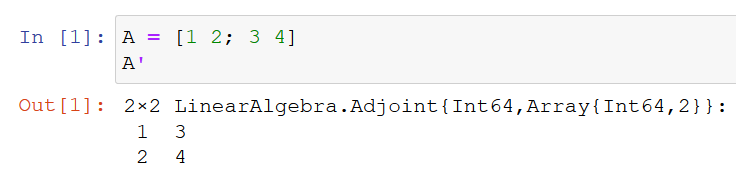Example 2:

 `# Defining a retangular matrix of size (2, 3) ` `B ``=` `[``1` `2` `3``; ``4` `5` `6``]    ` ` `  `# Transpose of B ` `B'                    `

Output: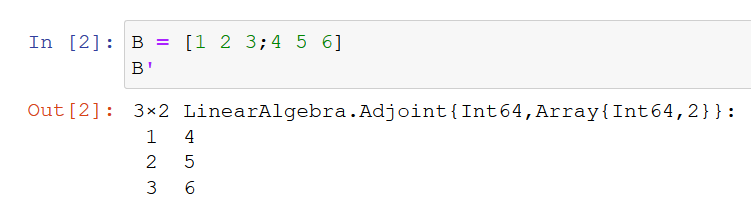#### Flipping a matrix: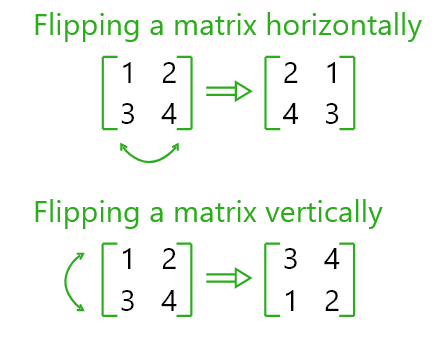• A matrix in Julia can be flipped via the X-axis i.e. horizontally or via the Y-axis i.e. vertically.
• To flip the matrix we use `reverse(< matrix >, dims= < 1 or 2 >))` 1 = vertically, 2 = horizontally.

Example 1: Flipping vertically

 `# Defining a rectangular matrix of size (2, 3) ` `B ``=` `[``1` `2` `3``; ``4` `5` `6``]     ` ` `  `# Flipping the matrix vertically ` `reverse(B, dims ``=` `1``)    `

Output: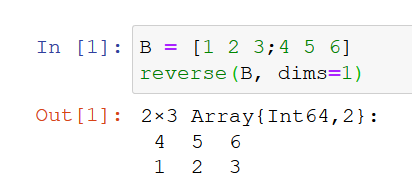Example 2: Flipping horizontally

 `# Flipping the matrix horizontally ` `reverse(B, dims ``=` `2``)    `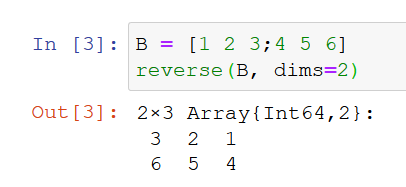#### Concatenating matrices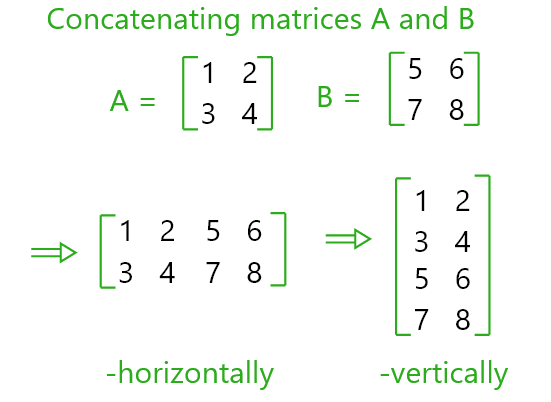• In Julia we can concatenate a matrix to another matrix to the right side of the initial matrix or to the bottom of it.
• We use `vcat(A, B)` to concatenate to the side.
• And `hcat(A, B)` to concatenate to the bottom.
• While concatenating to the side, we need to make sure that both the matrices have same number of rows.
• While concatenating to the bottom, we need to make sure that both the matrices have same number of columns.

Example 1: Concatenate to the side

 `# Creating a square matrix of size (2, 2) ` `A ``=` `[``1` `2``; ``3` `4``]         ` ` `  `# Creating a rectangular matrix of size (2, 3) ` `B ``=` `[``5` `6` `7``; ``8` `9` `10``]    ` `hcat(A, B) `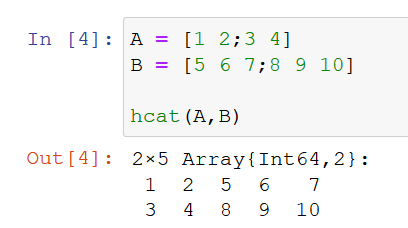Example 2: Concatenate to the bottom

 `# Creating a square matrix of size (3, 2) ` `A ``=` `[``1` `2``;``3` `4``; ``5` `6``]         ` ` `  `# Creating a rectangular matrix of size (4, 2) ` `B ``=` `[``5` `7``;``8` `9``; ``10` `11``;``14` `16``]    ` `vcat(A, B) `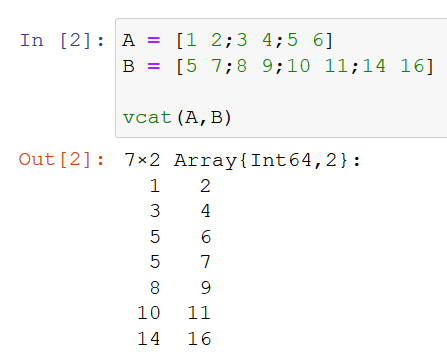#### Reshaping a matrix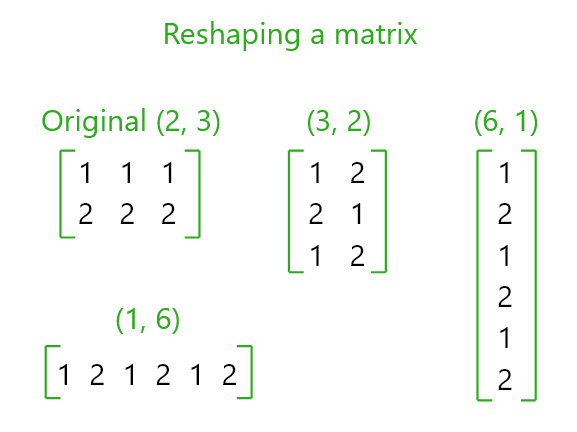We can reshape a matrix into another matrix of different size.
Example 1: Reshaping a matrix

 `# The original matrix with size (3, 2) ` `A ``=` `[``1` `2``; ``3` `4``; ``5` `6``]     `

• Output:
•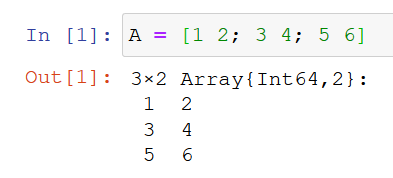Reshaping the matrix to size (2, 3)

 `reshape(A, (``2``, ``3``)) `

Output: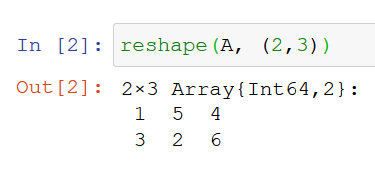Reshaping the matrix to size (6, 1)

 `reshape(A, (``6``, ``1``)) `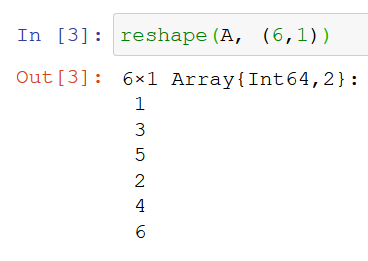Reshaping the matrix to size (1, 6)

 `reshape(A, (``1``, ``6``)) `

Output: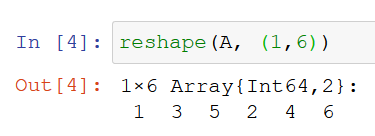#### Inverse of a matrix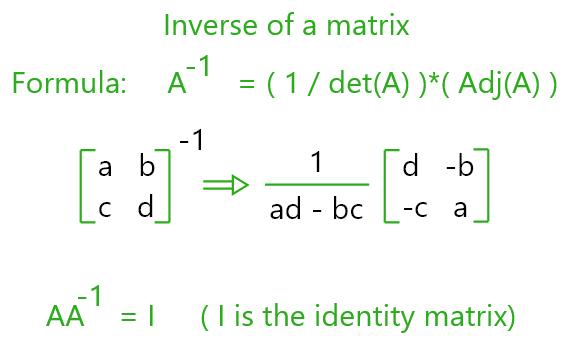• If A is a square matrix its multiplicative inverse is called its inverse matrix. Denoted by A-1.
• In Julia we use `inv(A)` to get the inverse of the matrix A.
• Example 1: Getting the Inverse of a matrix

 `# Creating a square matrix of size (2, 2) ` `A ``=` `[``4` `7``; ``2` `6``]       ` ` `  `# Getting the inverse of matrix A   ` `inv(A)                `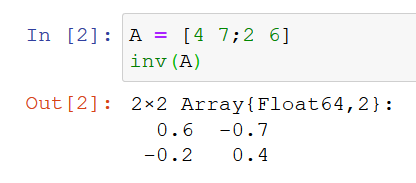Example 2: Getting Identity Matrix

 `# Creating a square matrix of size (2, 2) ` `A ``=` `[``4` `7``; ``2` `6``]      ` ` `  `# Getting the Identity matrix ` `A ``*` `inv(A)          `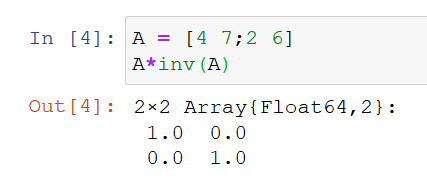My Personal Notes arrow_drop_up
Recommended Articles
Page :